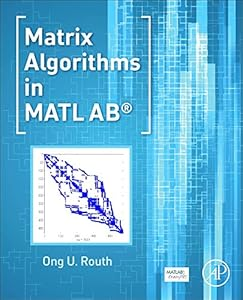# Matrix Algorithms in MATLAB9780128038048

Matrix Algorithms in MATLAB
by: Ong U. Routh
ISBN-10 书号： 0128038047
ISBN-13 书号： 9780128038048
Edition 版次： 1
Release Finelybook 出版日期： 2016-04-12
pages 页数： 478

Description
Matrix Algorithms in MATLAB focuses on the MATLAB code implementations of matrix algorithms. The MATLAB codes presented in the book are tested with thousands of runs of MATLAB randomly generated matrices,and the notation in the book follows the MATLAB style to ensure a smooth transition from formulation to the code,with MATLAB codes discussed in this book kept to within 100 lines for the sake of clarity. View more >
Key Features
Presents the first book available on matrix algorithms implemented in real computer code
Provides algorithms covered in three parts,the mathematical development of the algorithm using a simple example,the code implementation,and then numerical examples using the code
Allows readers to gain a quick understanding of an algorithm by debugging or reading the source code
Includes downloadable codes on an accompanying companion website,www.matrixalgorithmsinmatlab.com,that can be used in other software development
Primary: Researchers working in numerical methods; Engineers who utilize matrix computations; Secondary: graduate students taking a course on matrix computations
Contents
Chapter 1 Introduction
Chapter 2 Matrix Decomposition by Non-orthogonal Transformation
Chapter 3 Matrix Decomposition by Orthogonal Transformation
Chapter 4 Direct Algorithms of Solution of Linear Equations
Chapter 5 Iterative Algorithms of Solution of Linear Equations
Chapter 6 Direct Algorithms of Unsymmetric Eigenvalue Problem
Chapter 7 Direct Algorithms of Symmetric Eigenvalue Problem
Chapter 8 Iterative Algorithms of Eigenvalue Problem
Chapter 9 Algorithms of Singular Value Problem

Matrix Algorithms in MATLAB focuses on the MATLAB code implementations of matrix algorithms. The MATLAB codes presented in the book are tested with thousands of runs of MATLAB randomly generated matrices,and the notation in the book follows the MATLAB style to ensure a smooth transition from formulation to the code,with MATLAB codes discussed in this book kept to within 100 lines for the sake of clarity.
The book provides an overview and classification of the interrelations of various algorithms,as well as numerous examples to demonstrate code usage and the properties of the presented algorithms. Despite the wide availability of computer programs for matrix computations,it continues to be an active area of research and development. New applications,new algorithms,and improvements to old algorithms are constantly emerging.
Presents the first book available on matrix algorithms implemented in real computer code
Provides algorithms covered in three parts,the mathematical development of the algorithm using a simple example,the code implementation,and then numerical examples using the code
Allows readers to gain a quick understanding of an algorithm by debugging or reading the source code
Includes downloadable codes on an accompanying companion website,www.matrixalgorithmsinmatlab.com,that can be used in other software development

## 下载地址：

Matrix Algorithms in MATLAB 9780128038048.pdf

### 评论 抢沙发

#### 支付宝扫一扫打赏#### 微信扫一扫打赏